The Law Of Sines is something I memorized in a class once, but didn’t internalize: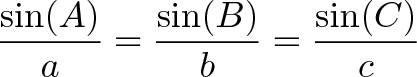$\displaystyle{\frac{\sin(A)}{a} = \frac{\sin(B)}{b} = \frac{\sin(C)}{c} }$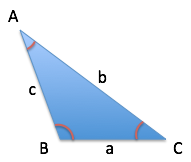Ok, that’s a neat connection, and maybe we can prove it by drawing some right triangles (of course) and re-arranging terms.

But what does it mean?

Rather than the Law of Sines, think of the Law of Equal Perspectives: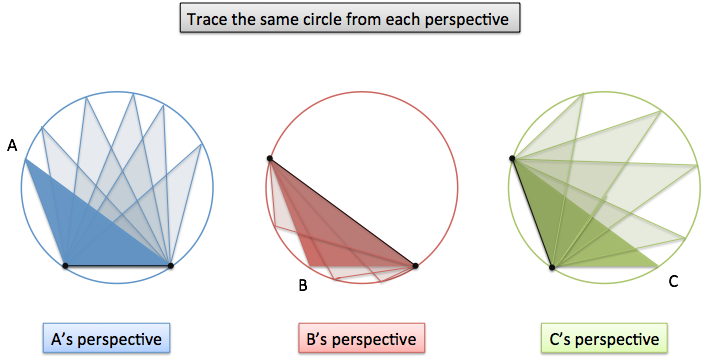Each angle & side can independently find the circle that wraps up the whole triangle. This connection lets us start with one angle and work out facts about the others.

## Analogy: Kids Describing A Monster

I occasionally frighten the neighborhood children by unchaining the mutant gorilla in my front yard.

The kids run screaming, telling different stories of what they’ve seen:

“Alice claims the monster was 20 feet tall, but we all know she exaggerates by doubling. And Billy’s a bit of a crybaby, and said it was 30 feet tall. Charlie’s fairly no-nonsense and said the beast was exactly 10 feet high.”

If we know a kid’s “exaggeration factor” and the size they claim, we can deduce the true size of the monster. (Furious George has a name, you know.)

Even better, we can predict what other kids might have said: If Alice claimed it was 40 feet, what would Charlie have said?

## Triangles And The Monster Circle

What do kids running from monsters have to do with triangles? Well, every triangle is trapped inside its own Monster Circle:Whatever triangle we draw, there’s some circle trying to gobble it up (technically, “circumscribe it”). Try this page to explore an example on your own.

Now here’s the magic: just knowing a single angle and its corresponding side, we can figure out the Monster Circle.

Here’s how. Let’s say we have a triangle like this: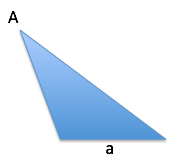We don’t know anything except the angle $A$ (call it 30 degrees) and the length of side $a$ (call it an inch).

First off: is this the correct drawing of the triangle? Probably not! We don’t know the other sides, so this is equally valid: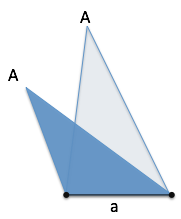It still has the same angle ($A$ = 30 degrees) and the size of the base hasn’t changed (still one inch).

What if we start drawing more possibilities?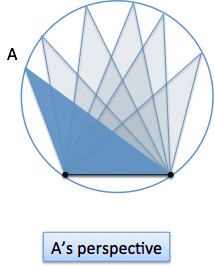Whoa. From $A$’s point of view all the possible triangles that have “$A$=30 degrees, $a$=1 inch” are on this circle. Whatever B and C end up being, they need to pick an option from this circle.

Similarly, we can argue this from the other perspectives:

• We can lock down angle $B$ and side $b$, and trace out a circle of possibilities
• We can lock down angle $C$ and side $c$, and trace out a circle of possibilitiesThis is the meaning of the Law of Sines: each angle unknowingly generates the same circle as the others.

(How do we prove, not just see that the possibilities lie on a circle? That’s the Inscribed Angle Theorem, for another day.)

## Calculating The Actual Size

We’ve figured out that there is a Monster Circle, now let’s see how big it is. Um… how?

Remember, we can slide around the circle and keep $A$ (30 degrees) and $a$ (1 inch) the same. So let’s slide until we make a right triangle: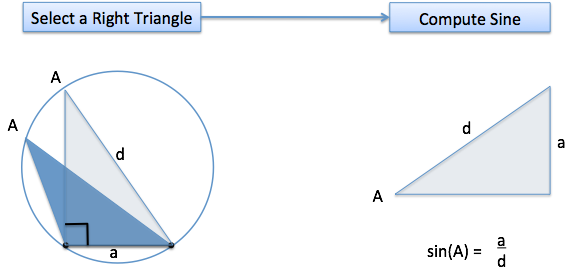Ah! Now we can use sine. Remember that sine is the percentage height compared to the max possible. The max possible height is the full diameter ($d$) of the Monster Circle.

(Why is a 90-degree angle across from the full diameter? Draw a square inside the circle, touching the sides. It must be symmetric, the diagonals pass through the center along the diameter, and are opposite a 90-degree angle.)

With a little re-arranging, we get: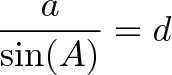$\displaystyle{ \frac{a}{\sin(A)} = d }$

Using the same logic for the other sides, we get: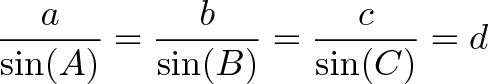$\displaystyle{ \frac{a}{\sin(A)} = \frac{b}{\sin(B)} = \frac{c}{\sin(C)} = d }$

In a way, $\sin(A)$ is the “exaggeration factor” that converts the size the angle measured ($a$) to the full diameter ($d$). Each angle is a different kid, and some really misjudge the size of the full circle based on what they see. (90-degrees is right on target.)

## Practice Problem

In our example above, $A$ is 30 degrees and $a$ is 1 inch.

We can calculate the diameter pretty fast. First, we get the sine: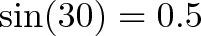$\displaystyle{\sin(30) = 0.5}$

That means our length $a$ is 50% of the max height, so the full diameter must be 2 inches.

This isn’t enough to figure out the triangle by itself. Let’s say angle $B$ comes along and says it is 45 degrees. How long is $b$?

Well,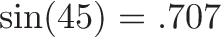$\displaystyle{\sin(45) = .707}$

which means that $b$ is .707 of the max diameter. Therefore,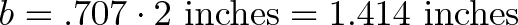$\displaystyle{ b = .707 \cdot \text{2 inches} = \text{1.414 inches}}$

Previously, I would plug numbers into the Law of Sines formula and chug away algebraically. Now I can think in terms of the Monster Circle: “Ok, I have the max diameter. I take the sine, and get the fraction of the max diameter for that side.”

Most books write the formula with sin(A) in the numerator. It might read better “Sine A over A” but it distorts the conclusion that $\frac{a}{\sin(A)}$ is the size of the circle.

Put the concept in your own words. The “Law of Sines” is a generic description of what’s in the formula, but the “Law of Equal Perspectives” explains what it means:

• All parts of the triangle have a perspective on the whole
• Sine is the “exaggeration factor” that scales up an individual side to the full diameter. (Sine is the percentage of the max possible, and we divide by it.)

Happy math.

## Appendix: Obtuse Angles

Technically, because $B$ is over 90 degrees, we can’t ever spin it and have either $A$ or $C$ be a right angle (if we could, the triangle would have over 180 degrees).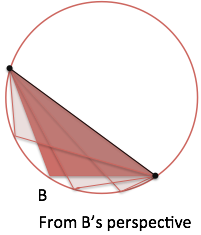What to do? Realize the 180-degree complement of $B$ (call it $B\star$) acts like a stand-in on the other side: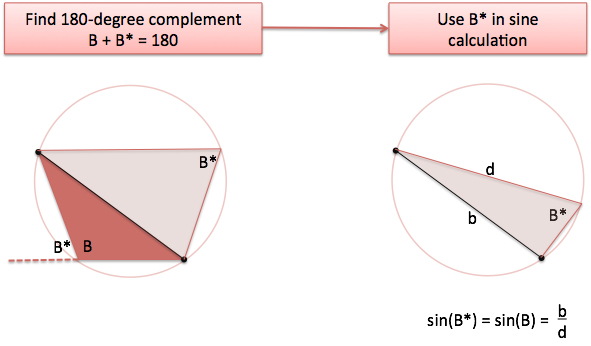$B \star$ has the same sine as $B$, which should make sense: they both point upwards along the same trajectory. To help us sleep better at night, we start with $B \star$ in the right-angle setup: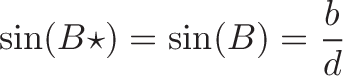$\displaystyle{\sin(B \star) = \sin(B) = \frac{b}{d} }$

and get to the same conclusion as before. Phew.

However, the fact that $B$ and $B\star$ can be swapped can lead to problems.

If I have a triangle where I know $A$ (30 degrees) and $a$ (1 inch), and then say $b$ is 1.5 inches, what can you deduce?

The max diameter is 2 inches as before, so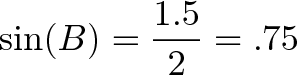$\displaystyle{\sin(B) = \frac{1.5}{2} = .75}$

Unfortunately, there are two angles with that sine value: a calculator says $\sin^{-1}(.75) = 48$ degrees, but $180 – 48 = 132$ degrees would work too (more details).

Also, the triangle may not be possible given a hypothetical scenario. If I say $b$ is 3 inches, you know something’s amiss. The max diameter was already calculated to be 2. Even a 90-degree angle, the best possible, could only have a side of 2 inches.

Diagram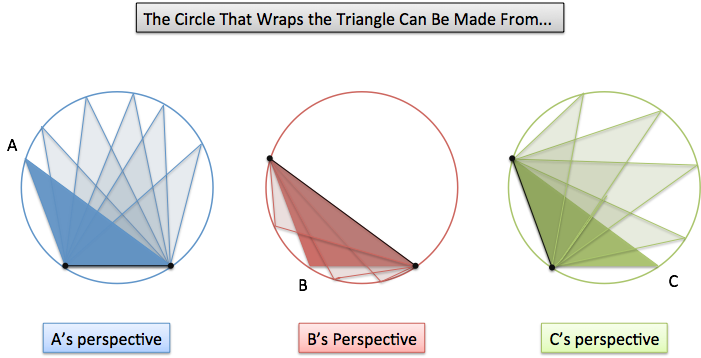Example Suppose $A$=30 and $a$=1 inch. Since $\sin(A) = 0.5$, the Monster Circle is 1 / 0.5 = 2 inches wide. Given another angle, I can figure out the length of its side. If $B$ = 45 degrees, then side $b$ takes up $\sin(45) = .707$ of the diameter, and is 1.414 inches.
Technical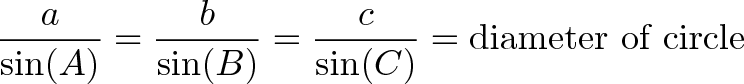$\displaystyle{\frac{a}{\sin(A)} = \frac{b}{\sin(B)} = \frac{c}{\sin(C)} = \text{diameter of circle} }$